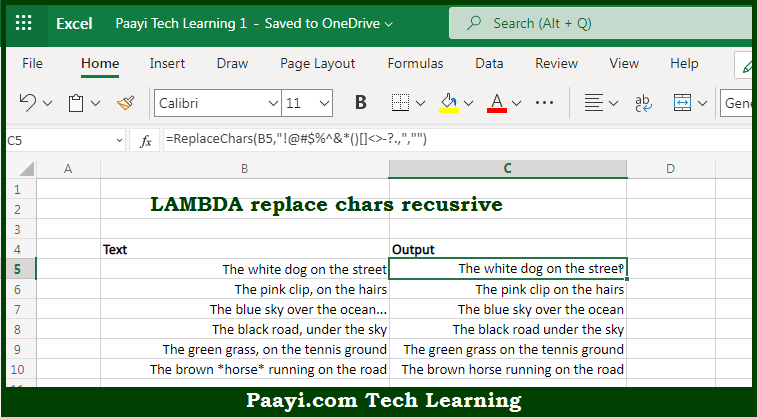# Learn How to LAMBDA Replace Characters Recursive in Microsoft Excel

Written by | 0 Comments | 573 Views

In this article, you will learn how to evaluate things in Dynamic Arrays with formulas in Microsoft Excel using a single/combination(s) of functions. You will also know How to LAMBDA Replace Characters Recursive and see the generic formula.

Learn How to LAMBDA Replace Characters Recursive  in Microsoft Excel

The main purpose of this formula is to create a custom function to replace characters. Here we will learn how to replace characters recursive in the given data range in the workbook in Microsoft Excel. That implies, with the help of a formula based on the LAMBDA function you can able to create a custom function to replace characters. So, with the help of this formula, you can able to replace characters recursive in the given data range in the workbook in Microsoft Excel.

General Formula to LAMBDA Replace Characters Recursive

=LAMBDA(str,chars,sub,IF(chars="",str, ReplaceChars(SUBSTITUTE(str,LEFT(chars),sub),MID(chars,2,LEN(chars)-1),sub)))

The Explanation for LAMBDA Replace Characters RecursiveSo we know that with the help of the given formula above you can able to replace characters. Here we will learn how to replace characters recursive in the given data range in the workbook in Microsoft Excel. As we know that the LAMBDA function can be used to create custom, reusable functions. Here we are illustrating the feature called recursion, in which a function calls itself. Recursion can be used to create elegant, compact, non-redundant code. So, with the help of this formula, you can able to replace characters. Here we will learn how to replace characters recursive in the given data range in the workbook in Microsoft Excel.